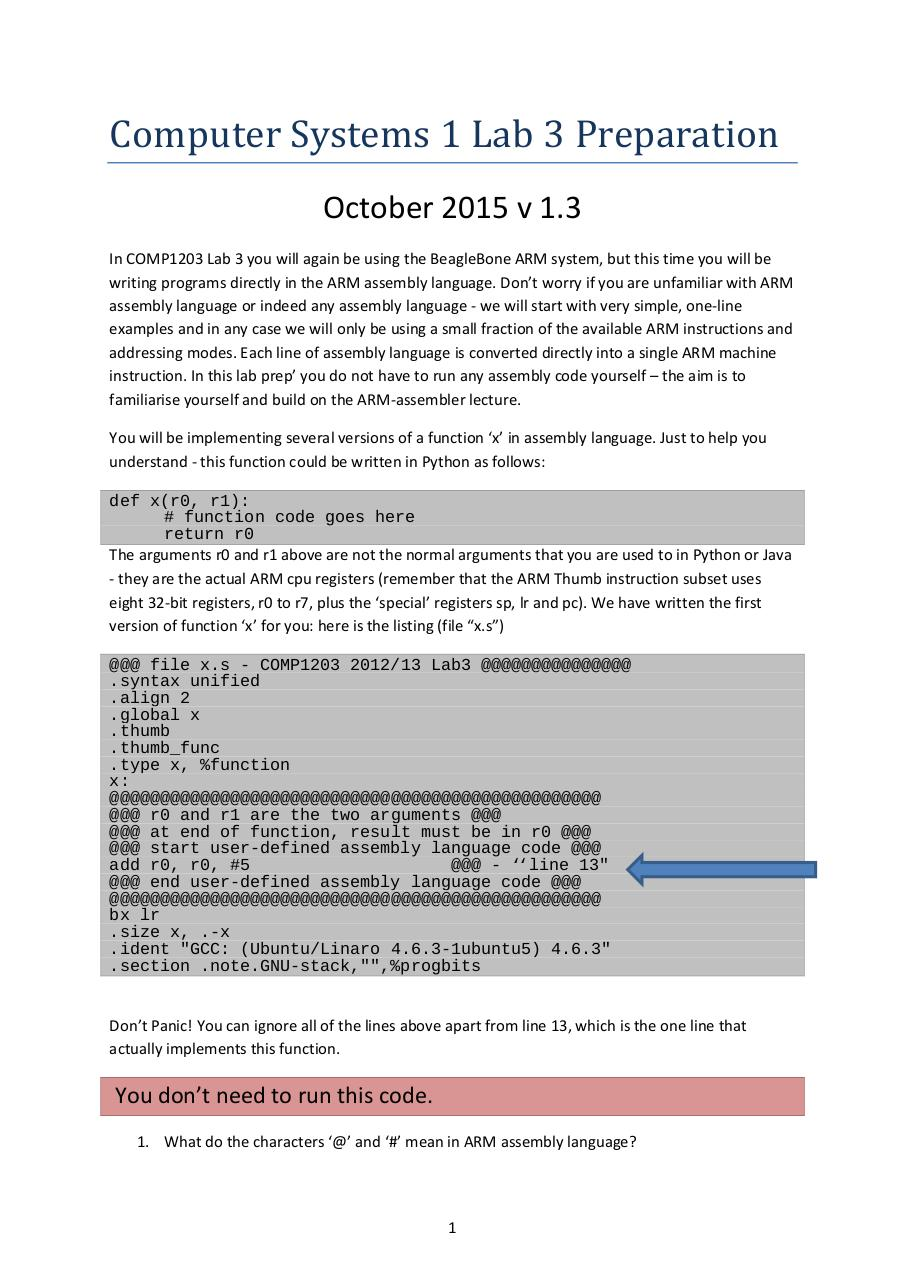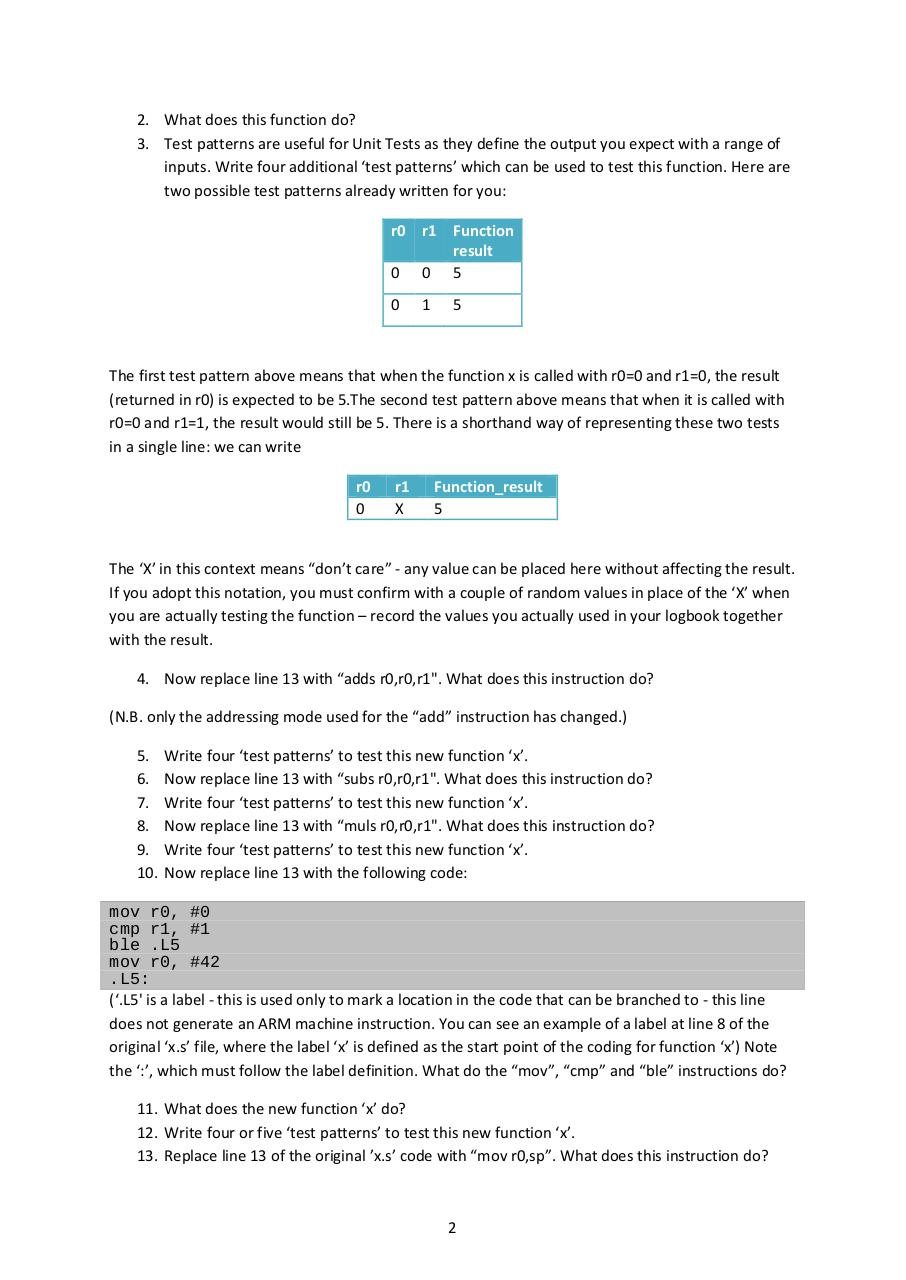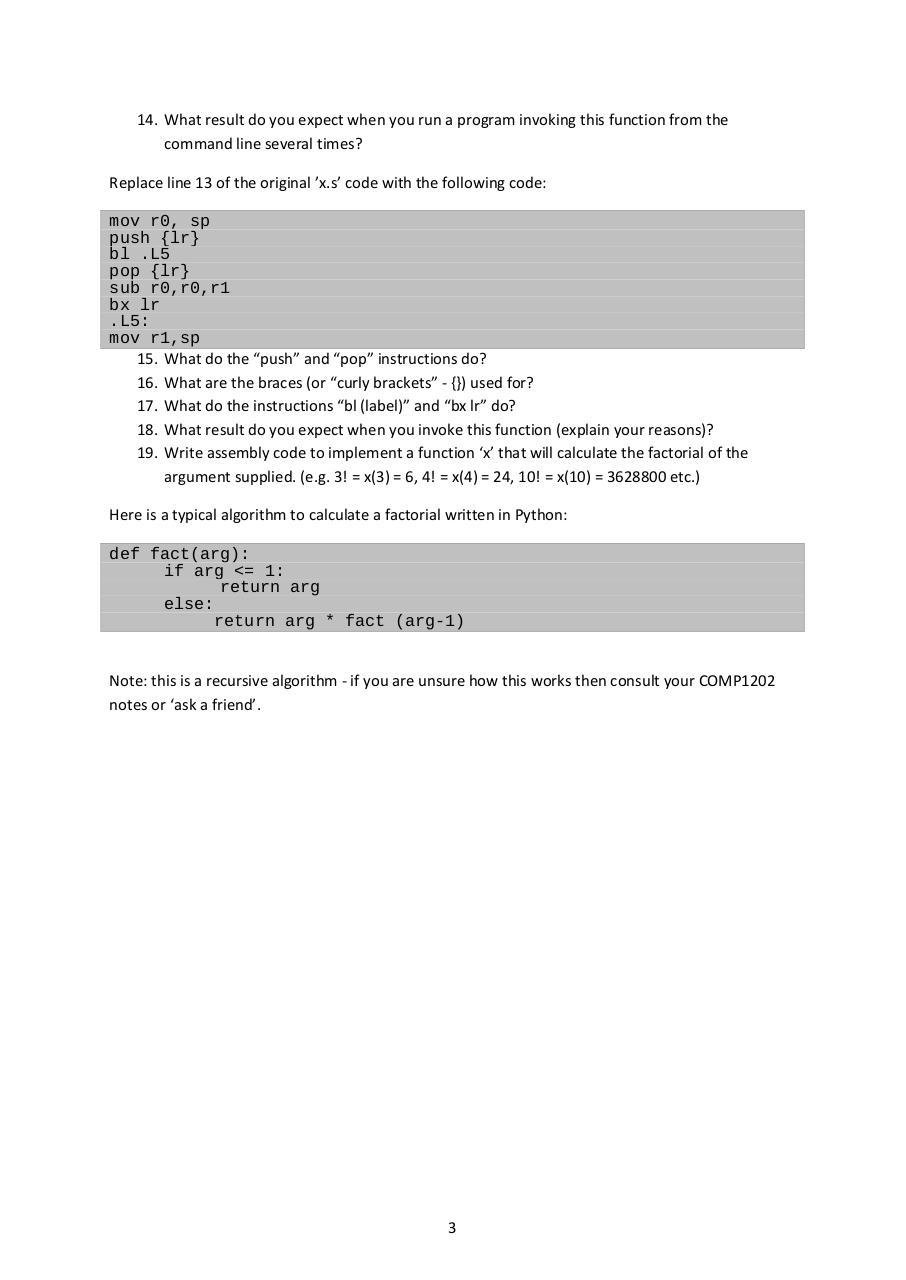# lab3prep .pdf

### File information

Original filename: lab3prep.pdf
Title: Comp1203 Lab1 practical
Author: kirk

This PDF 1.5 document has been generated by Acrobat PDFMaker 11 for Word / Adobe PDF Library 11.0, and has been sent on pdf-archive.com on 17/11/2015 at 14:01, from IP address 46.246.x.x. The current document download page has been viewed 820 times.
File size: 107 KB (3 pages).
Privacy: public file

lab3prep.pdf (PDF, 107 KB)

### Document preview

Computer Systems 1 Lab 3 Preparation
October 2015 v 1.3

In COMP1203 Lab 3 you will again be using the BeagleBone ARM system, but this time you will be
writing programs directly in the ARM assembly language. Don’t worry if you are unfamiliar with ARM
assembly language or indeed any assembly language - we will start with very simple, one-line
examples and in any case we will only be using a small fraction of the available ARM instructions and
addressing modes. Each line of assembly language is converted directly into a single ARM machine
instruction. In this lab prep’ you do not have to run any assembly code yourself – the aim is to
familiarise yourself and build on the ARM-assembler lecture.
You will be implementing several versions of a function ‘x’ in assembly language. Just to help you
understand - this function could be written in Python as follows:
def x(r0, r1):
# function code goes here
return r0
The arguments r0 and r1 above are not the normal arguments that you are used to in Python or Java
- they are the actual ARM cpu registers (remember that the ARM Thumb instruction subset uses
eight 32-bit registers, r0 to r7, plus the ‘special’ registers sp, lr and pc). We have written the first
version of function ‘x’ for you: here is the listing (file “x.s”)
@@@ file x.s - COMP1203 2012/13 Lab3 @@@@@@@@@@@@@@@
.syntax unified
.align 2
.global x
.thumb
.thumb_func
.type x, %function
x:
@@@@@@@@@@@@@@@@@@@@@@@@@@@@@@@@@@@@@@@@@@@@@@@@@
@@@ r0 and r1 are the two arguments @@@
@@@ at end of function, result must be in r0 @@@
@@@ start user-defined assembly language code @@@
@@@ - ‘‘line 13"
@@@ end user-defined assembly language code @@@
@@@@@@@@@@@@@@@@@@@@@@@@@@@@@@@@@@@@@@@@@@@@@@@@@
bx lr
.size x, .-x
.ident "GCC: (Ubuntu/Linaro 4.6.3-1ubuntu5) 4.6.3"
.section .note.GNU-stack,"",%progbits
Don’t Panic! You can ignore all of the lines above apart from line 13, which is the one line that
actually implements this function.

You don’t need to run this code.
1. What do the characters ‘@’ and ‘#’ mean in ARM assembly language?

1

2. What does this function do?
3. Test patterns are useful for Unit Tests as they define the output you expect with a range of
inputs. Write four additional ‘test patterns’ which can be used to test this function. Here are
two possible test patterns already written for you:
r0 r1 Function
result
0 0 5
0

1

5

The first test pattern above means that when the function x is called with r0=0 and r1=0, the result
(returned in r0) is expected to be 5.The second test pattern above means that when it is called with
r0=0 and r1=1, the result would still be 5. There is a shorthand way of representing these two tests
in a single line: we can write
r0
0

r1
X

Function_result
5

The ‘X’ in this context means “don’t care” - any value can be placed here without affecting the result.
If you adopt this notation, you must confirm with a couple of random values in place of the ‘X’ when
you are actually testing the function – record the values you actually used in your logbook together
with the result.
4. Now replace line 13 with “adds r0,r0,r1". What does this instruction do?
(N.B. only the addressing mode used for the “add” instruction has changed.)
5.
6.
7.
8.
9.
10.

Write four ‘test patterns’ to test this new function ‘x’.
Now replace line 13 with “subs r0,r0,r1". What does this instruction do?
Write four ‘test patterns’ to test this new function ‘x’.
Now replace line 13 with “muls r0,r0,r1". What does this instruction do?
Write four ‘test patterns’ to test this new function ‘x’.
Now replace line 13 with the following code:

mov r0, #0
cmp r1, #1
ble .L5
mov r0, #42
.L5:
(‘.L5' is a label - this is used only to mark a location in the code that can be branched to - this line
does not generate an ARM machine instruction. You can see an example of a label at line 8 of the
original ‘x.s’ file, where the label ‘x’ is defined as the start point of the coding for function ‘x’) Note
the ‘:’, which must follow the label definition. What do the “mov”, “cmp” and “ble” instructions do?
11. What does the new function ‘x’ do?
12. Write four or five ‘test patterns’ to test this new function ‘x’.
13. Replace line 13 of the original ’x.s’ code with “mov r0,sp”. What does this instruction do?

2

14. What result do you expect when you run a program invoking this function from the
command line several times?
Replace line 13 of the original ’x.s’ code with the following code:
mov r0, sp
push {lr}
bl .L5
pop {lr}
sub r0,r0,r1
bx lr
.L5:
mov r1,sp
15. What do the “push” and “pop” instructions do?
16. What are the braces (or “curly brackets” - {}) used for?
17. What do the instructions “bl (label)” and “bx lr” do?
18. What result do you expect when you invoke this function (explain your reasons)?
19. Write assembly code to implement a function ‘x’ that will calculate the factorial of the
argument supplied. (e.g. 3! = x(3) = 6, 4! = x(4) = 24, 10! = x(10) = 3628800 etc.)
Here is a typical algorithm to calculate a factorial written in Python:
def fact(arg):
if arg &lt;= 1:
return arg
else:
return arg * fact (arg-1)
Note: this is a recursive algorithm - if you are unsure how this works then consult your COMP1202

3